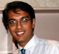# Modelling a Noisy Communication Signal in MATLAB for the Analog to Digital Conversion ProcessA critical thing to realize while modeling the signal that is going to be digitally processed is the SNR. In a receiver, the noise floor (hence the noise variance and hence its power) are determined by the temperature and the Bandwidth. For a system with a constantbandwidth, relatively constant temperature, the noise power remains relatively constant as well. This implies that the noise variance is a constant.

In MATLAB, the easiest way to create a noisy signal is by using awgn(sig,SNR,'measured'). Or, this has been the method that I have used extensively to generate a noisy signal. Earlier, I failed to realize that this was not correctly modeling the signal that I wanted to process. Using awgn will produce noise after measuring the signal power.

Let me explain with an example. Say that we have generated a signal in MATLAB with variance of 0.5. Suppose we wanted SNR of 0dB, in MATLAB we would simply have y = awgn(sig,0,'measured').

If we measured the var(sig-y) we would end up with an answer of 0.5.

Let us follow the rest of the explanation in the pdf attachedThe following short MATLAB script generates the noisy signal:

% Inputs: SNR Value, the Variance of the Noise of the reciever and the

% original sig

% Outputs: The noisy signal and the noise with the required noise variance

function [sign, noise] = sig_model(SNR, noise_var, sig)

sig_std = std(sig);

y = sqrt(noise_var)*sqrt(10^(SNR/10))*sig/sig_std;

sig_variance = var(sig)

scaled_signal_variance = var(y)

sign = awgn(y,SNR,'measured');

noise = sign-y;

noise_variance = var(noise)

Above script will generate the model of the analog signal that will hit the front end of the ADC. In the next blog, I will investigate the effects of the ADC process on this noisy signal.

[ - ]
Comment by October 30, 2007Hello, I am a practicing DSP engineer with interest in communications. I dont currently work in a communications field, but I am taking night classes in an MSEE program in this topic. I have some comments / questions: I am not accustomed to thinking of signal power as being equal to its variance, so I have been exploring this concept. Examining the defintions (for real signals): Average Power = 1/N * summation as n:1->N, of s(n)^2. Variance = 1/N * summation as n:1->N, of [s(n)-mean(s)]^2 These two only become equal when the signal's mean is zero. Would you say that this is typically (or always?) the case in Communications signals? Thanks!
[ - ]
Comment by October 25, 2015Hi,
Look for Guassian Distribution and related stuff. As u r doing MSEE, for Communication there is a prereq. "Random Variables and Stochastic Processes" , that deals with all ur questions, mean, variance, etc.
What u said is true if a signal is distorted by Guassian noise, that is usually the case and a presumption in your case
[ - ]
Comment by February 17, 2008u r doing nice work. am also working on project based on matlab. My aim is to reduce noise from noisy signal,image,audio &video signal.can u help me
[ - ]
Comment by February 17, 2008ashonadvance, I am wondering what your SNR is and over what bandwidth. What bandwidths are the actual signals you are looking to reduce noise on. The simples way to do this, if you are operating over a large bandwidth with a "narrow band" signal, is to simply band pass filter it. This can increase your SNR quite a bit.
[ - ]
Comment by June 12, 2008HEY! im working on the transmitter and channel part of an SDR using matlab,could u help with the codin part?my knowledge of dsp is limited.just finished my 2nd yr b.tech
[ - ]
Comment by April 9, 2009hello sir....am just an uder grad working on real time adaptive noise cancellation using neural networks and am stuck....!! any help...?? the problem is knw the basic idea of noise cancellation by substraction but inserting neurals into it seems to be tough...n am not able to find matlab simulation function either....expecting a hellp from ur end...thanks....
[ - ]
Comment by October 8, 2009i need engineers help on image processing alogorithm developement
[ - ]
Comment by October 19, 2009hi vakil sir...am doing post grad,i need your help,i doing a dissertation on automatic gain control for a wireless receiver,in this main aim is to keeping the signal of wireless receiver within limits to avoid saturation of the ADC.as i have limited knowledge on MAT Lab,could u plz help me...i am expecting positive result from you sir.i am waiting for ur reply.
[ - ]
Comment by July 19, 2010hi..i just want to ask u about SNR..how we want to measuring SNR using matlab for image processing?any source code suitable with that?thanks
[ - ]
Comment by September 3, 2010hello, can u send me some matlab code for noise filterring in speeech signal.bcz i am doing project under noice filterring by using fragmentation algorithms.E-mail: manoj.siripuram@gmail.com thank u .
[ - ]
Comment by September 13, 2010Hello , i have some doubts can you answer that plz 1)Difference between Windowing And Segmentation? 2)How do We find Feature Vector âs From each frame? 3)How can We Choose Length of Feature Vector? 4)How do we find Energy of Speech Using Signal Representation?Is any thing to do change rather than Signal represantation? Thank you
[ - ]
Comment by January 17, 2011good material
[ - ]
Comment by April 25, 2011good,thks

To post reply to a comment, click on the 'reply' button attached to each comment. To post a new comment (not a reply to a comment) check out the 'Write a Comment' tab at the top of the comments.